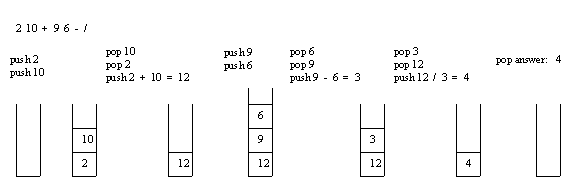# Evaluation of Postfix Expression in C [Algorithm and Program]

Here you will get algorithm and program for evolution of postfix expression in C.

In postfix or reverse polish notation, every operator follows all of its operands.

For example: 5 3 2 * +

Also Read: Infix to Postfix Conversion in C [Program and Algorithm]

## Algorithm for Evaluation of Postfix Expression

Create an empty stack and start scanning the postfix expression from left to right.

• If the element is an operand, push it into the stack.
• If the element is an operator O, pop twice and get A and B respectively. Calculate BOA and push it back to the stack.
• When the expression is ended, the value in the stack is the final answer.

Evaluation of a postfix expression using a stack is explained in below example:## Program for Evaluation of Postfix Expression in C

Output

Enter the expression(eg: 59+3*)
Single digit operand and operators only:74+5-

Value of expression=6

### 9 thoughts on “Evaluation of Postfix Expression in C [Algorithm and Program]”

1.What is the option for TWO DIGIT OPERANDS ??

1.#include
#include

int n, top = -1, *stack;

void push(int x){
if(top==n) return;
stack[++top]=x;
}

int pop(){
if(top==-1) return -1;
return stack[top–];
}

int peek(){
if(top==-1) return -1;
return stack[top];
}

void display(){
for(int i=top ; i>-1 ; i–) printf(“%d “,stack[i]);
printf(“\n\n”);
}

int main(){

n = 10;

printf(“Initializing the stack with size 10\n\n”);

stack = (int*)malloc(n*sizeof(int));

printf(“Pushing elements into the stack\n1\n2\n3\n\n”);

push(1);
push(2);
push(3);

printf(“Displaying elements of the stack -\n”);

display();

printf(“The top of the stack = %d\n\n”,peek());

printf(“Pop the top of the stack = %d\n\n”,pop());

printf(“Pop the top of the stack = %d\n\n”,pop());

printf(“Displaying elements of the stack -\n”);

display();

return 0;
}

2.can you write a program for infix to postfix transformation and its evaluation in one program

1.ill send u tomorrow

2.#include
#include
#include

#define SIZE 40

int pop();
void push(int);

char postfix[SIZE];
int stack[SIZE], top = -1;

int main()
{
int i, a, b, result, pEval;
char ch;

for(i=0; i<SIZE; i++)
{
stack[i] = -1;
}
printf("\nEnter a postfix expression: ");
scanf("%s",postfix);

for(i=0; postfix[i] != '\0'; i++)
{
ch = postfix[i];

if(isdigit(ch))
{
push(ch-'0');
}
else if(ch == '+' || ch == '-' || ch == '*' || ch == '/')
{
b = pop();
a = pop();

switch(ch)
{
case '+': result = a+b;
break;
case '-': result = a-b;
break;
case '*': result = a*b;
break;
case '/': result = a/b;
break;
}

push(result);
}

}

pEval = pop();

printf("\nThe postfix evaluation is: %d\n",pEval);

return 0;
}

void push(int n)
{
if (top -1)
{
n = stack[top];
stack[top–] = -1;
return n;
}
else
{
printf(“Stack is empty!\n”);
exit(-1);
}
}

1.i believe you missed the pop function can you please update it as i need the code asap

3.3.4.